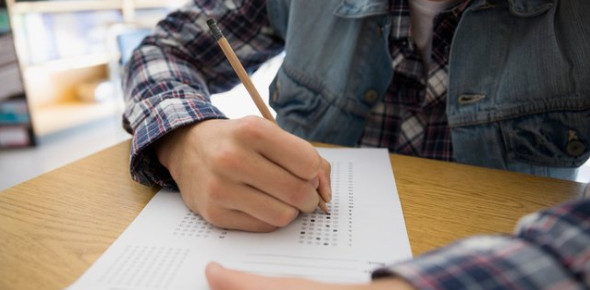# Plan Pre-Act Exam: Trivia Quiz!

10 Questions | Total Attempts: 105SettingsThe PLAN program is an exam administered by ACT, Inc. , to 10th graders in preparation for the real ACT exam that they will take in their high school career. The exam allows 10th graders to measure their college readiness and their ability to take standardized tests.

• 1.
A car averages 27 miles per gallon. If gas costs \$4.04 per gallon, which of the following is closest to how much the gas would cost for this car to travel 2,727 typical miles?
• A.

\$44.44

• B.

\$109.08

• C.

\$118.80

• D.

\$444.40

• E.

\$408.04

• 2.
Abandoned mines frequently fill with water. Before an abandoned mine can be reopened, the water must be pumped out. The size of the pump required depends on the depth of the mine. If pumping out a mine that is D feet deep requires a pump that pumps a minimum of  + 4D – 250 gallons per minute, pumping out a mine that is 150 feet deep would require a pump that pumps a minimum of how many gallons per minute?
• A.

800

• B.

500

• C.

362

• D.

1750

• E.

1250

• 3.
The length, in inches, of a box, is 3 inches less than twice its width, in inches. Which of the following gives the length, l inches, in terms of the width, w inches, of the box?
• A.

L = 2w — 3

• B.

L = w — 3

• C.

L = w + 3

• D.

L = 2w + 3

• 4.
When x = 3 and y = 5, by how much does the value of 3x2 – 2exceed the value of 2x2 – 3y?
• A.

50

• B.

20

• C.

16

• D.

4

• E.

14

• 5.
What is the value of x when 2x + 3 = 3x – 4 ?
• A.

1/5

• B.

-1/5

• C.

-7

• D.

1

• E.

7

• 6.
In quadrilateral PQRS below, sides PS and QR are parallel for what value of x?
• A.

132

• B.

158

• C.

120

• D.

110

• E.

70

• 7.
What is the greatest common factor of 42, 126, and 210?
• A.

2

• B.

6

• C.

14

• D.

21

• E.

42

• 8.
Sales for a business were 3 million dollars more the second year than the first, and sales for the third year were double the sales for the second year. If sales for the third year were 38 million dollars, what were sales, in millions of dollars, for the first year?
• A.

16

• B.

17.5

• C.

20.5

• D.

22

• E.

35

• 9.
A typical high school student consumes 67.5 pounds of sugar per year. As part of a new nutrition plan, each member of a track team plans to lower the sugar he or she consumes by at least 20% for the coming year. Assuming each track member had consumed sugar at the level of a typical high school student and will adhere to this plan for the coming year, what is the maximum number of pounds of sugar to be consumed by each track team member in the coming year?
• A.

14

• B.

44

• C.

48

• D.

54

• E.

66

• 10.
In the figure below, ray was constructed starting from rays and . By using a compass D and G were marked equidistant from E on rays  and . The compass was then used to locate a point F, distinct from E, so that F is equidistant from D and G. For all constructions defined by the above steps, the measures of ∠DEF and ∠GEF:
• A.

Are equal.

• B.

Are NOT equal.

• C.

Sum to 30°.

• D.

Sum to 45°.

• E.

Sum to 60°.

Related TopicsBack to top